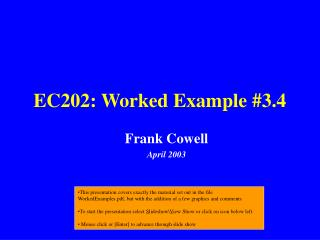DownloadDownload PresentationEC202: Worked Example #3.4

# EC202: Worked Example #3.4

Download Presentation## EC202: Worked Example #3.4

- - - - - - - - - - - - - - - - - - - - - - - - - - - E N D - - - - - - - - - - - - - - - - - - - - - - - - - - -
##### Presentation Transcript

1. EC202: Worked Example #3.4 Frank Cowell April 2003 • This presentation covers exactly the material set out in the file WorkedExamples.pdf, but with the addition of a few graphics and comments • To start the presentation select Slideshow\View Show or click on icon below left. • Mouse click or [Enter] to advance through slide show

2. WX3.4: Monopoly and competition Part 1: Competition

3. WX3.4: Find Firm’s Supply Curve Integrate MC in the question to get total costs Divide by Q to get average costs Differentiate to find minimum AC at Where average costs are: Given a price can then find output from supply curve

4. Q*= P - a —— b WX3.4: The Firm’s Supply Curve marginal cost a+bQ supply curve P P average cost F/Q+a+0.5bQ Q Q

5. WX3.4: Monopoly and competition Part 2: Unregulated monopoly

6. WX3.4: Find monopolist’s equilibrium Given the demand curve (AR), total revenue is So, MR is FOC for the monopolist (MR=MC) is Solving for Q we get And from this we have

7. WX3.4: Monopolist’s equilibrium P a+bQ marginal cost F/Q+a+0.5bQ P** average cost c** average revenue A - 0.5bQ A - bQ marginal revenue Q** Q

8. WX3.4: Monopoly and competition Part 3: Regulated monopoly

9. WX3.4: Introduce price ceiling A price ceiling alters the effective demand curve So AR is now: Multiply by Q and then differentiate to get MR: Note that MR is discontinuous, exactly where AR is kinked Effect of price ceiling depends on position of MC relative to this discontinuity

10. WX3.4: effect of high price ceiling P (Output unchanged) marginal cost Pmax P** c** average revenue marginal revenue Q0 Q** Q

11. WX3.4: effect of low price ceiling P (Output falls) marginal cost P** Pmax c** average revenue marginal revenue Q0 Q** Q

12. WX3.4: effect of intermediate price ceiling P (Output rises to Q0) marginal cost P** Pmax c** average revenue marginal revenue Q0 Q** Q

13. WX3.4: intermediate price ceiling (2) P (Output rises) marginal cost P** Pmax c** average revenue marginal revenue Q0 Q** Q

14. WX3.4: Points to remember • Make good use of a helpful diagram to “see” the problem • Re-use the solution to one part of the problem to build the next. • Don’t be fazed by the presence of a discontinuity – everything is nice and regular either side of it.